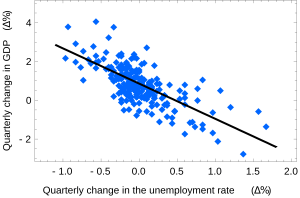# Ordinary least squares facts for kids

Kids Encyclopedia FactsOkun's law in macroeconomics states that in an economy the GDP growth should depend linearly on the changes in the unemployment rate. Here the ordinary least squares method is used to construct the regression line describing this law. The observed values are shown in blue, the expected ones are shown in black.

Ordinary least squares or linear least squares is a method for estimating unknown parameters in statistics. It is a method used in linear regression. The goal of the method is to minimize the difference between the observed responses and the responses predicted by the linear approximation of the data. A smaller difference means that model fits the data better. Ordinary least squares is a special case of a method commonly called least squares. The resulting estimator can be expressed by a simple formula.

## Images for kidsOrdinary least squares Facts for Kids. Kiddle Encyclopedia.